# Test Prep Plan - Take a practice test

Take this practice test to check your existing knowledge of the course material. We'll review your answers and create a Test Prep Plan for you based on your results.
How Test Prep Plans work
1
2Based on your results, we'll create a customized Test Prep Plan just for you!
3Study smarter
Study more effectively: skip concepts you already know and focus on what you still need to learn.

# FTCE Math: Limits Chapter Exam

Exam Instructions:

Choose your answers to the questions and click 'Next' to see the next set of questions. You can skip questions if you would like and come back to them later with the yellow "Go To First Skipped Question" button. When you have completed the practice exam, a green submit button will appear. Click it to see your results. Good luck!

### Page 1

#### Question 1 1. Calculate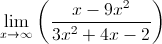#### Question 2 2. Which of the following is equivalent to the statement below?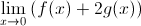#### Question 3 3. Calculate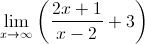#### Question 4 4. The expression below can also be written as: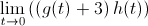#### Question 5 5. The ceiling function is graphically translated as a stepwise function. What is the limit of f(x) = ceil(x) as x approaches 4.5?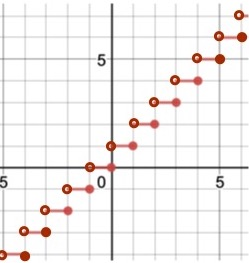### Page 2

#### Question 6 6. Using the given data, predict the likely value of the continuous function z.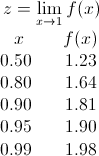#### Question 8 8. The expression below can also be written as: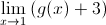#### Question 10 10. Using the graph, find the value of z, then add 1.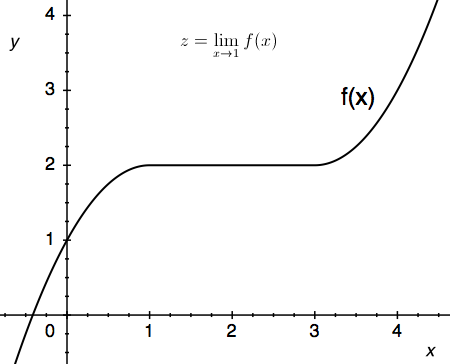### Page 3

#### Question 12 12. Use the graph to solve for z.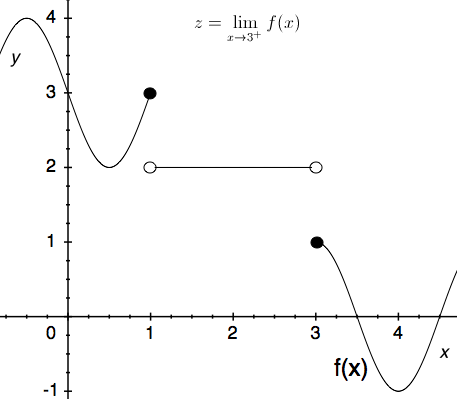#### Question 13 13. The floor function is graphically represented as a stepwise function. What is the limit of f(x) = floor(x) as x approaches 4?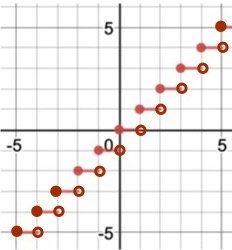#### Question 14 14. Solve for the limit: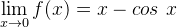#### Question 15 15. Use the given formula to solve for z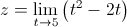### Page 4

#### Question 17 17. Use the graph to solve for z.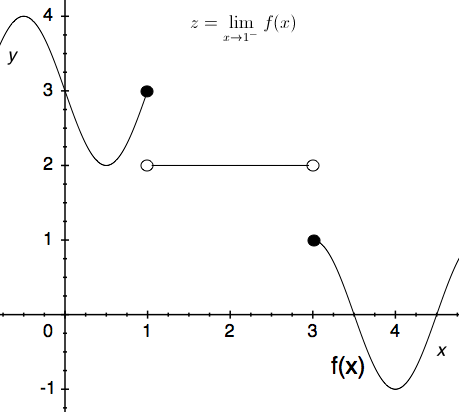#### Question 18 18. Calculate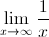#### Question 19 19. Calculate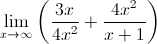### Page 5

#### Question 21 21. Use the given graph and formula to solve for z.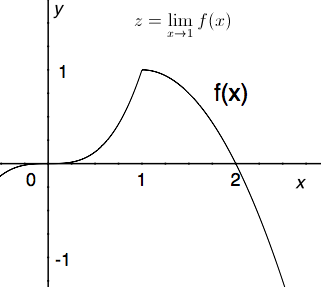#### Question 22 22. Calculate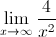#### Question 23 23. Which of the following is true?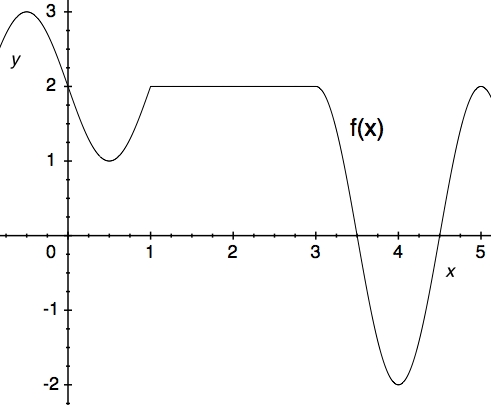#### Question 24 24. Use the given graph and formula to solve for z.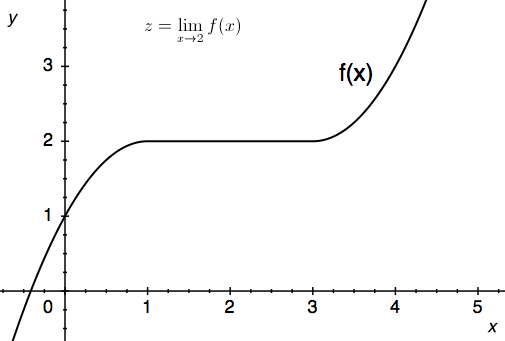#### Question 25 25. Use the graph to solve for z.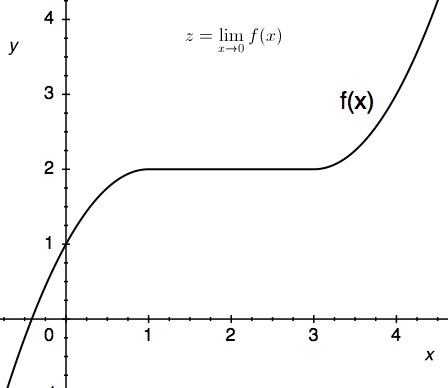### Page 6

#### Question 27 27. Evaluate the limit below.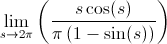#### Question 28 28. Evaluate the limit below.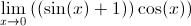#### Question 29 29. Using the given data, solve for the continuous function y.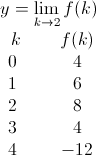#### Question 30 30. Use the graph to solve for z.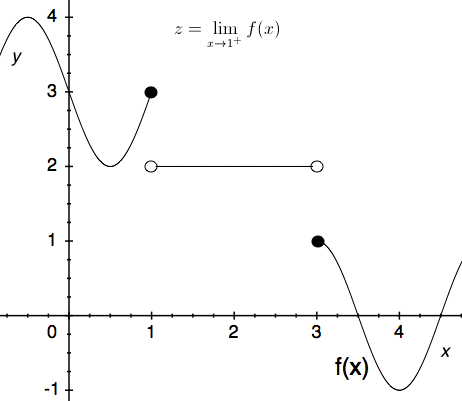#### FTCE Math: Limits Chapter Exam Instructions

Choose your answers to the questions and click 'Next' to see the next set of questions. You can skip questions if you would like and come back to them later with the yellow "Go To First Skipped Question" button. When you have completed the practice exam, a green submit button will appear. Click it to see your results. Good luck!

Support